## Zeke’s car had an initial velocity os 12 m/sec slows down to 2 m/sec in 4.5 seconds. What is the acceleration of Zeke’s car? *

Question

Zeke’s car had an initial velocity os 12 m/sec slows down to 2 m/sec in 4.5 seconds. What is the acceleration of Zeke’s car? *

in progress 0
2 months 2021-07-30T08:02:13+00:00 1 Answers 0 views 0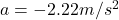Explanation:

the information we have is:

initial velocity: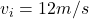final velocity: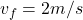and the time: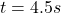We need to find the acceleration of the car.

For this we use formula to calculate the acceleration given the information we were given: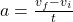acceleration is equal to the difference between the final velocity and the initial velocity divided by time.

We substitute all the known values: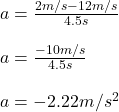the acceleration is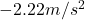the result is negative because the car is slowing down.